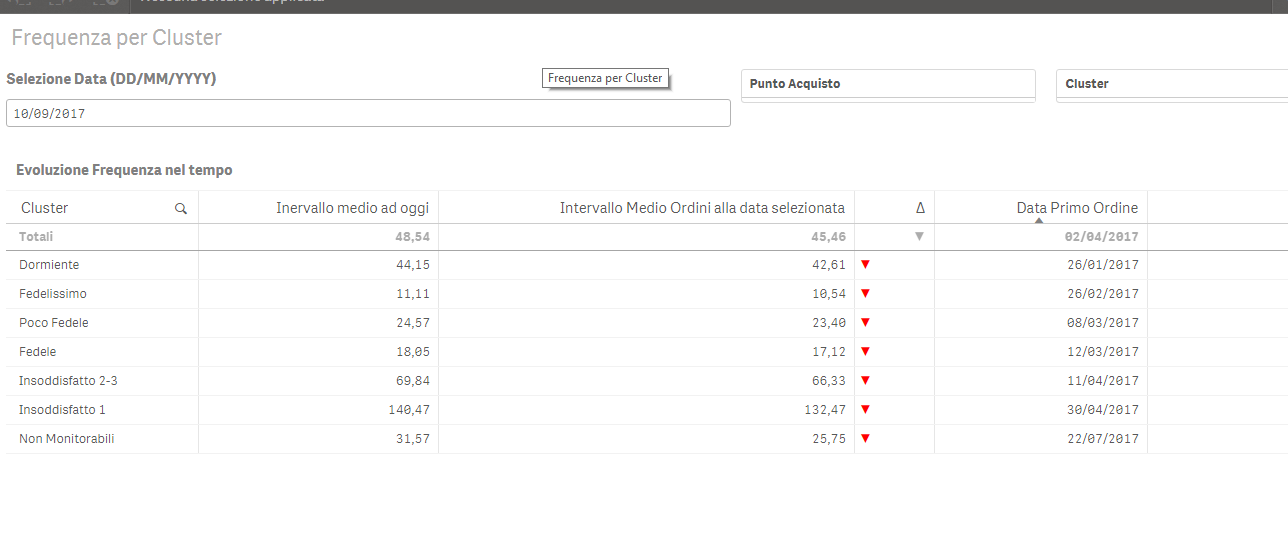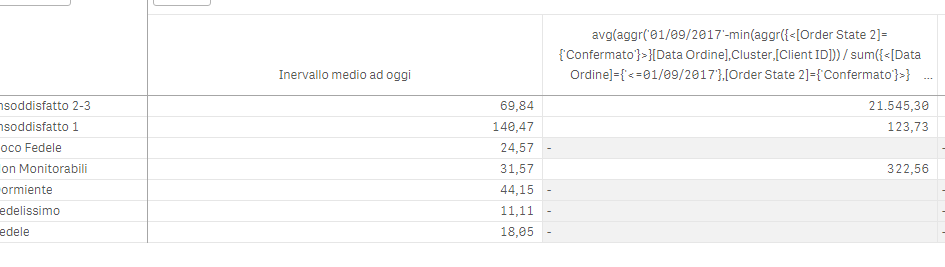# New to Qlik Sense

Discussion board where members can get started with Qlik Sense.

Announcements
BARC’s The BI Survey 19 makes it official. BI users love Qlik. GET REPORT
Highlighted
New Contributor III

## avg(aggr())

Hi everybody, I need an help: I'm working on a qliksense document. I need to create a table with Cluster's Average range to today and to another day choose by user and then growth or decrease with green or red symbol. .

I can't use the right way to do this. I found a way to calculate Client's Average range

I attach the qliksense app and I hope Someone can help me,

Thank You AnnaTags (3)
9 RepliesPartner

## Re: average interval

Hi Anna,

What's exactly the issue ?

I took a look at your document, I tried to put September 10 as date but I don't understand the chart object and column involved.

New Contributor III

## Re: average interval

Hi Andrea,

My problem is that i can't measure 'average interval' for all client's cluster because this measure is only for single client. i can't find a diffeent solution.You have to look the columns 'Average Interval at Today' and 'Average interval at selected date'. This is what you should see by entering September 10.

! !Partner

## Re: average interval

what's the expected results for the two columns ?

and also how those columns should be calculated ?

New Contributor III

## Re: average interval

I want to calculate the average order interval for each cluster. The Middle Order Range at today column is fine because I did an average of the Interval Media field while I can not calculate the Middle Order Range at selected date column using the formula Data selezionata – data primo ordine /Numero Totale  because for every cluster i have too many min(data ordine) .  The expected results  is like this but with only cluster dimension:New Contributor III

## Re: average interval

I have to exspand IntervalloMedioOrdiniCliente to the entire customer's cluster.

This is the part of script where i calculate the field:

NumeroOrdiniRif:

NoConcatenate

[Client ID],

sum([Numero Ordini]) as [Numero Ordini per Cliente],

(Today()-min([Data Ordine])) as gg,

max([Data Ordine]) as maxData,

min([Data Ordine]) as minData,

(Today()-min([Data Ordine]))/sum([Numero Ordini]) as IntervalloMedioOrdiniCliente

Resident Ordine_T

where [Order State 2] = 'Confermato'

group by [Client ID];

New Contributor III

## Re: average interval

I need to calculate the avarege for field intervallo medio alla data selezionata:

I used this formula but it doesn't work:

avg(aggr(if(isnull(column(1))=0 and ('\$(vMeasure2)'-min({<[Order State 2]={'Confermato'}>}[Data Ordine]))

/sum({<[Data Ordine]={'<=\$(vMeasure2)'},[Order State 2]={'Confermato'}>}[Numero Ordini])>=0,

('\$(vMeasure2)'-min({<[Order State 2]={'Confermato'}>}[Data Ordine]))

/sum({<[Data Ordine]={'<=\$(vMeasure2)'},[Order State 2]={'Confermato'}>}[Numero Ordini]))),Cluster)Partner

## Re: average interval

try using this expression in first table:

avg(

aggr(

avg( ( '\$(vMeasure2)'-minData )

/

[Numero Ordini per Cliente] ), Cluster, [Client Name] )

)

and the below for the same measure in second table:

avg( ( '\$(vMeasure2)'-minData )

/

[Numero Ordini per Cliente])

if you select a single cluster they should have the same value.

New Contributor III

## Re: average interval

Andrea sei italiano? Ho provato in questo modo ma non funziona:

avg(aggr('\$(vMeasure2)'-min(aggr({<[Order State 2]={'Confermato'}>}[Data Ordine],Cluster,[Client ID]))

/

sum({<[Data Ordine]={'<=\$(vMeasure2)'},[Order State 2]={'Confermato'}>}[Numero Ordini]),Cluster,[Client ID]))Partner

## Re: average interval

sì ho dato un'occhiata ma ci vorrebbe ancora più tempo.